# An Introduction to Luminescence Quantum Yields

The Photoluminescence Quantum Yield (PLQY) of a material or molecule is defined as the quantity of photons emitted as a fraction of the quantity of photons absorbed. This characteristic of a fluorescent or fluorophore molecule is critical for comprehending molecule behavior and interactions within a range of important materials.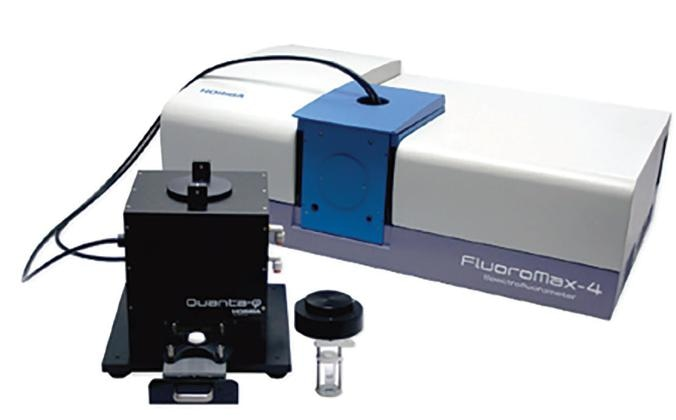Figure 1. An integrating sphere fiber-coupled to a fluorometer for PLQY measurements.

Similarly, a molecule’s Electroluminescence Quantum Yield (ELQY) is defined as the number of photons emitted by the electron current of a particular device. This measurement is essential in categorizing and monitoring display devices, lighting or other photovoltaic materials.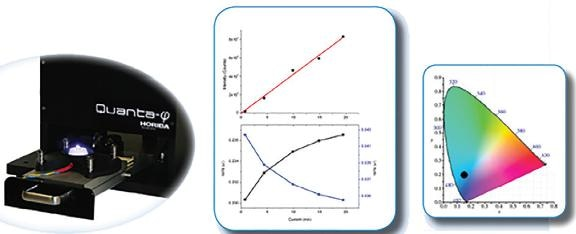Figure 2. Electroluminescence can be measured using an integrating sphere (left) by fitting a powered device such as an LED into the sample tray. Center: The integrated intensity can be measured with input voltage or current. Right: Color can be plotted in CIE 1931 coordinates by measurement of the spectrum in the sphere.

PLQY and ELQY are commonly used with:-

• Novel nanomaterials
• Nanoparticles
• Solar cells and photovoltaics
• Graphene or Single Walled Carbon nanotubes
• Quantum dots
• Coordination chemistry
• Lighting and display materials such as LEDs and OLEDs
• Electrovoltaics
• Films and coatings
• Paintings, colorimetry and coatings
• Cured and doped polymers, gels and hydrogels

Three methods are employed in order to measure PLQY. These are:

• The comparison method
• Direct method (integrating sphere)

Each of these methods is explored in further detail, below.

## How Do I Use the Comparative Method to Determine Quantum Yield?

The comparative method makes use of a known reference standard – a sample with emission and absorbance properties that are close to the sample of interest and with a known PLQY value. Absorbance and fluorescence are measured for the reference standard, then the same measurements are undertaken for the sample being studied.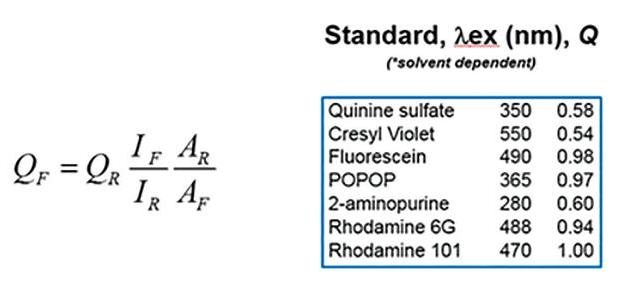Figure 3. Left: The equation for calculating the fluorescence quantum yield of an unknown (QF) by comparing it to the spectrum of a known standard. Right: A table of some known PLQY standards and their respective excitation wavelengths and quantum yields.

The equation below is used where QF is the quantum yield of an unknown fluorescent sample and QR is the quantum yield of the reference standard. Here, IF and IR are the integrated fluorescence intensities for the unknown and reference samples respectively, while AF and AR are the respective absorbance values of the unknown and reference samples.

The drawback to this method is that there exists a very limited amount of reference standards, so there are distinct limits to where this particular method can be applied.

## How Do I Use Fluorescence Lifetimes for Quantum Yield Determination?

Fluorescence lifetimes can be used alongside different concentrations of a quencher in order to calculate a molecule’s quantum yield.

The equation below is used where tf is the quantum yield, and kf, knr, and kt are the rate constants of fluorescence, non-radiative dissipation and energy transfer, respectively. τf is the fluorescence lifetime of the sample.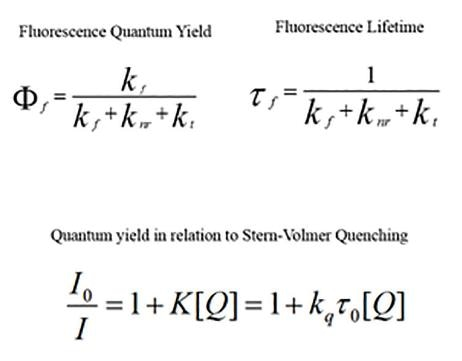Figure 4. Fluorescence Quantum yield equation calculated by the rate constants of fluorescence (kf), non-radiative dissipation (knr) and energy transfer (kt). Fluorescence lifetime calculated by one over the sum of the rate constants. And the quantum yield in relation to the Stern-Volmer quenching constant (K), the biomolecular quenching constant (kq) and the lifetime ( t0). (Lakowicz, 2006)

PLQY, in this instance, is determined by the rate constants of these non-radiative processes (for example Stern-Volmer quenching and FRET) as they compete with fluorescence.

The addition of a dilution series of quencher to a fluorescent solution allows for the calculation of PLQY by ascertaining the Stern-Volmer quenching constants (K) and the bimolecular quenching constant (kq).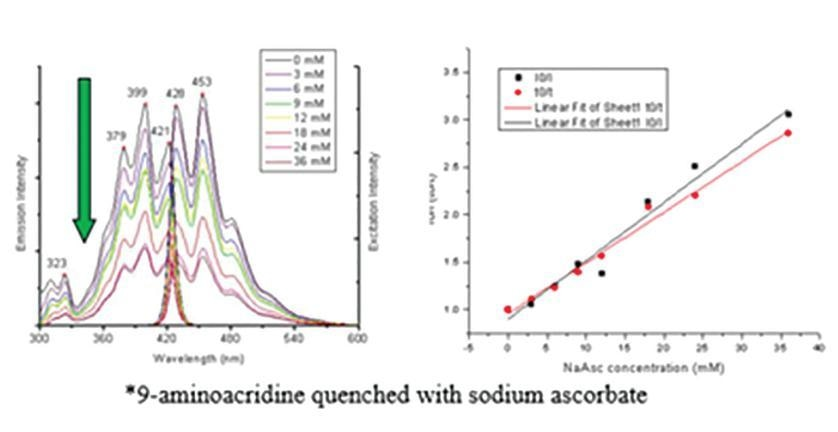Figure 5. Left: Fluorescence excitation and emission spectra for different concentrations of sodium ascorbate quenching fluorescence from 9-aminoacridine. Center: Frequency-domain lifetime by modulation and phase measurements (center) for the same solutions. Right: Lifetime and intensity ratios (I/I0 and t/t0) versus concentrations. Linear fits to these plots yield quenching constants.

This method is certainly robust, but it does require a considerable amount of sample preparation, making it especially inconvenient for solid samples.

## How Do I Use an Integrating Sphere for Quantum Yield Measurements?

The Integrating Sphere Method is a more direct method for measuring PLQY. With this technique, a sphere is coated with a reflective surface in order to capture all the light entering or leaving the sphere. This surface is generally made from barium sulfate-based materials or Spectralon®.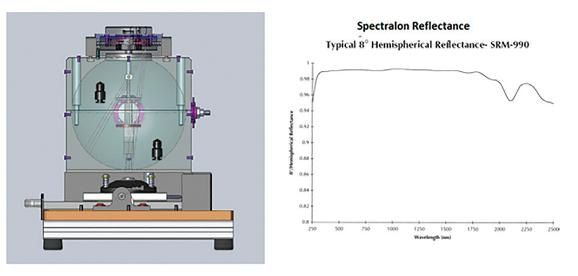Figure 6. Left: An integrating sphere where samples are placed on the inside of the sphere and then fluorescence is measured. Right: The reflectance spectrum of Spectralon® material that coats the inside of an integrating sphere. (LabSphere Spectralon(R) datasheet, 2017)

The fluorescence emission (Ec) and the scatter (Lc) of the sample are measured alongside the emission and scatter of a blank (La and Ea). These two spectral measurements (sample and blank) allow the PLQY to be directly calculated, as can be seen in the equation below.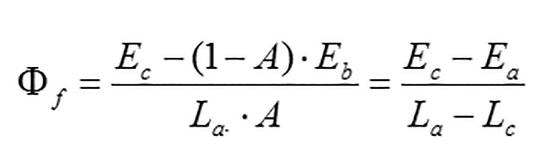Figure 7. Quantum yield (Ff) equation from measurement using an integration sphere.

In this example, Eb is the integrated luminescence from the sample caused by indirect luminescence from the sphere, while A is the absorbance of the sample at the excitation wavelength.

The PLQY and any associated error analysis is then calculated by incorporating the two traces with appropriate spectral correction factors.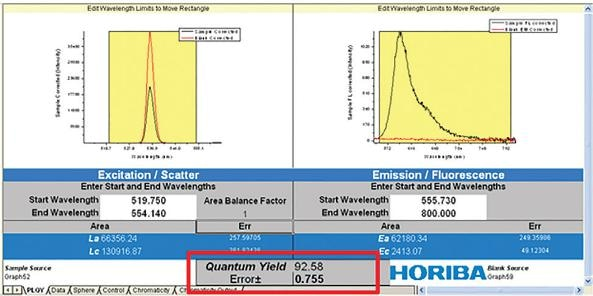Figure 8. Calculation of PLQY from scatter (left) and fluorescence (right) of blank and sample.This information has been sourced, reviewed and adapted from materials provided by HORIBA Scientific.

## Citations

• APA

HORIBA Scientific. (2020, January 24). An Introduction to Luminescence Quantum Yields. AZoM. Retrieved on November 26, 2020 from https://www.azom.com/article.aspx?ArticleID=16083.

• MLA

HORIBA Scientific. "An Introduction to Luminescence Quantum Yields". AZoM. 26 November 2020. <https://www.azom.com/article.aspx?ArticleID=16083>.

• Chicago

HORIBA Scientific. "An Introduction to Luminescence Quantum Yields". AZoM. https://www.azom.com/article.aspx?ArticleID=16083. (accessed November 26, 2020).

• Harvard

HORIBA Scientific. 2020. An Introduction to Luminescence Quantum Yields. AZoM, viewed 26 November 2020, https://www.azom.com/article.aspx?ArticleID=16083.